NCERT Solutions for Class 6 Maths Chapter 7 Fractions Ex 7.3 are part of NCERT Solutions for Class 6 Maths. Here we have given NCERT Solutions for Class 6 Maths Chapter 7 Fractions Ex 7.3.

 Board CBSE Textbook NCERT Class Class 6 Subject Maths Chapter Chapter 7 Chapter Name Fractions Exercise Ex 7.3 Number of Questions Solved 9 Category NCERT Solutions

## NCERT Solutions for Class 6 Maths Chapter 7 Fractions Ex 7.3

Question 1.
Write the fractions. Are all these fractions equivalent?
(a)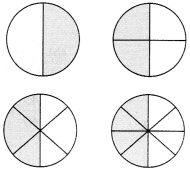(b)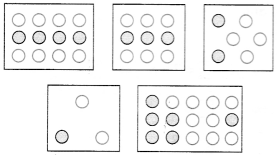Solution :
(a)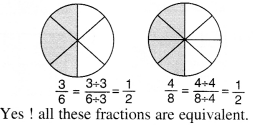(b)No ! all these fractions ae not equivalent.

Question 2.
Write the fractions and pair up the equivalent fractions from each row.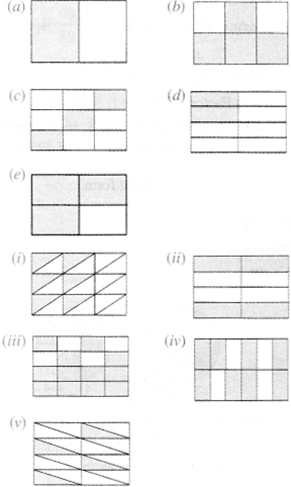Solution: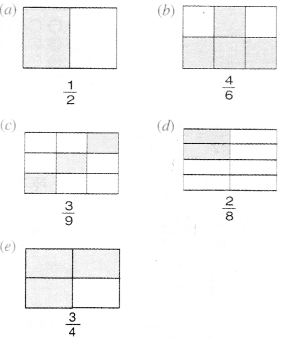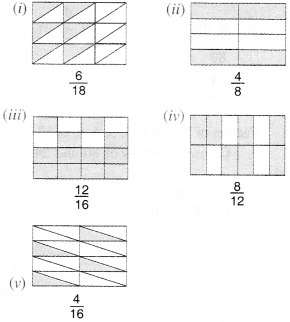Equivalent fractions are
(a), (ii);
(b), (,iv);
(c), (i);
(d), (v);
(e), (iii).

Question 3.
Replace in each of the following by the correct number: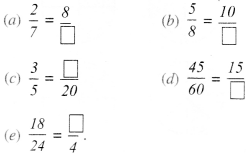Solution :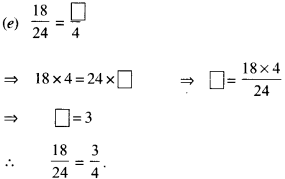Question 4.
Find the equivalent fraction of $$\frac { 3 }{ 5 }$$ having
(a) denominator 20
(b) numerator 9
(d) numerator 27
(c) denominator 30
Solution :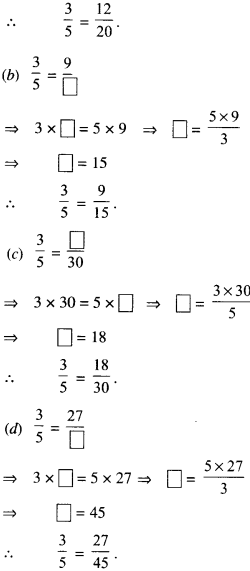Question 5.
Find the equivalent fraction of $$\frac { 36 }{ 48 }$$ with
(a) numerator 9
(b) denominator 4.
Solution :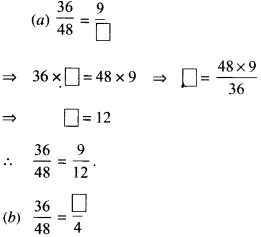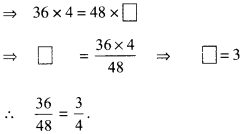Question 6.
Check whether the given fractions are equivalent :
(a) $$\frac { 5 }{ 9 }$$, $$\frac { 30 }{ 54 }$$
(b) $$\frac { 3 }{ 10 }$$, $$\frac { 12 }{ 50 }$$
(c) $$\frac { 7 }{ 13 }$$, $$\frac { 5 }{ 11 }$$
Solution :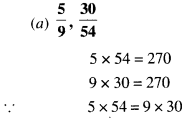∴ The given fractions $$\frac { 5 }{ 9 }$$ and $$\frac { 30 }{ 54 }$$ are equivalent.∴ The given fractions $$\frac { 3 }{ 10 }$$ and $$\frac { 12 }{ 50 }$$ are not equivalent.∴ The given fractions $$\frac { 7 }{ 3 }$$ and $$\frac { 5 }{ 11 }$$ are not equivalent.

Question 7.
Reduce the following fractions to simplest form: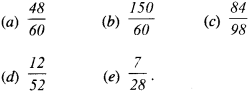Solution :
(a) $$\frac { 48 }{ 60 }$$
Factors of 48 are 1, 2, 3,4, 6, 8, 12, 16, 24 and 48.
Factors of 60 are 1, 2, 3,4, 5, 6, 10,12, 15, 20, 30 and 60.
∴ Common factors of 48 and 60 are 1, 2, 3, 4, 6 and 12. Highest of these common factors is 12.
∴ H.C.F. of 48 and 60 is 12.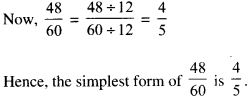(b) $$\frac { 150 }{ 60 }$$
Factors of 150 are 1,2,3,5,6.10,15,25,30,50, 75 and 150.
Factors of 60 are 1, 2. 3, 4. 5, 6, 10, 12, 15, 20, 30 and 60.
∴ Common factors of 150 and 60 are 1, 2, 3, 5,6, 10, 15 and 30.
Highest of these common factors is 30.
∴ H.C.F. of 150 and 60 is 30.(c) $$\frac { 84 }{ 98 }$$
Factors of 84 are 1, 2, 3,4, 6, 7, 12, 14, 21, 28, 42 and 84.
Factors of 98 are 1, 2, 7, 14,49 and 98.
∴ Common factors of 84 and 98 are 1,7 and 14. Highest of these common factors is 14.
∴ H.C.F. of 84 and 98 is 14.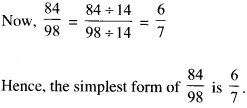(d) $$\frac { 12 }{ 52 }$$
Factors of 12 are 1, 2, 3,4, 6 and 12.
Factors of 52 are 1, 2, 4, 13, 26 and 52.
∴ Common factors of 12 and 52 are 1,2 and 4. Highest of these common factors is 4.
∴ H.C.F. of 12 and 52 is 4.(e) $$\frac { 7 }{ 28 }$$
Factors of 7 are 1 and 7.
Factors of 28 are 1, 2,4, 7, 14 and 28.
∴ Common factors of 7 and 28 are 1 and 7. Highest of these common factors is 7.
∴ H.C.F. of 7 and 28 is 7.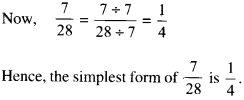Question 8.
Ramesh had 20 pencils, Sheelu had 50 pencils and Jamaal had 80 pencils. After 4 months, Ramesh used up 10 pencils, Sheelu used up 25 pencils and Jamaal used up 40 pencils. What fraction did each use up? Check if each has used up an equal fraction of his/her pencils?
Solution :
For Ramesh
Number of pencils he had = 20 Number of pencils used by him =10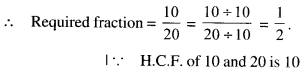For Sheelu
Number of pencils she had = 50 Number of pencils used by her = 25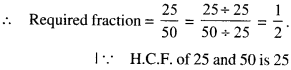For Jamaal
Number of pencils he had = 80 Number of pencils used by him = 40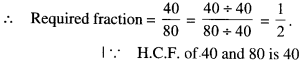Yes ! each has used up an equal fraction of his/her pencils.

Question 9.
Match the equivalent fractions and write another two more for each :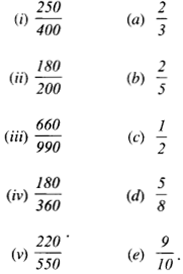Solution :
(i) $$\frac { 250 }{ 400 }$$
Factors of 250 are 1, 2, 5, 10, 25, 50, 125 and 250.
Factors of 400 are 1,2,4,5,8,10,16,20,25,40, 80, 100, 200 and 400.
∴ Common factors of 250 and 400 are 1,2,5, 10, 25 and 50.
Highest of these common factors is 50.
∴ H.C.F. of 250 and 400 is 50.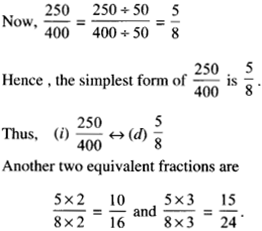(ii) $$\frac { 180 }{ 200 }$$
Factors of 180 are 1,2, 3,4, 5, 6,9,10,12,15, 18, 20, 30, 36, 45, 60, 90 and 180.
Factors of 200 are 1,2, 4, 5, 8, 10, 20, 25, 40, 50, 100, 200.
∴ Common factors of 180 and 200 are 1,2, 4, 5, 10 and 20.
Highest of these common factors is 20.
∴ H.C.F. of 180 and 200 is 20.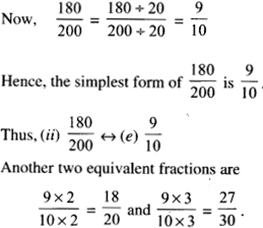(iii) $$\frac { 600 }{ 990 }$$
Factors of 660 are 1,2,3,4,5,6,10,12,22,30, 66, 110, 132, 165, 220, 330 amd 660.
Factors of 990 are 1,2,3,5,6,9,10,11,30,33, 90, 99, 110, 165, 198, 330, 495 and 990.
∴ Common factors of 660 and 990 are 1,2,3, 5,6, 10, 30, 110 and 330.
Highest of these common factors is 330.
∴ H.C.F. of 660 and 990 is 330.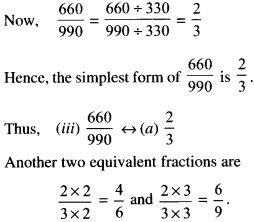(iv) $$\frac { 180 }{ 360 }$$
Factors of 180 are 1, 2, 3,4, 5, 6, 9, 10, 12,15, 18, 20, 30, 36,45, 60, 90 and 180.
Factors of 360 are 1, 2, 3,4, 5, 6,9,10, 12, 15, 18, 20, 24, 30, 36,40, 60, 72, 90, 120, 180 and 360.
∴ Common factors of 180 and 360 are 1,2, 3,4,5,6,9,10,12,15,18.20, 30, 36,60,90 and 180.
Highest of these common factors is 180.
∴ H.C.F. of 180 and 360 is 180.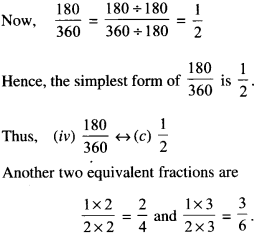(v) $$\frac { 220 }{ 550 }$$
Factors of 220 are 1,2,4, 5,10,11, 20, 22,44, 55, 110 and 220.
Factors of 550 are 1, 2, 5, 10, 22, 25, 55, 110, 275 and 550.
∴ Common factors of 220 and 550 are 1,2,5, 10, 20 and 110.
Highest of these common factors is 110.
∴ H.C.F. of 220 and 550 is 110.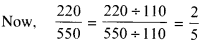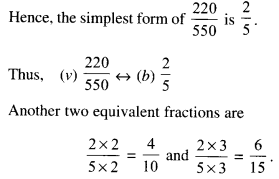We hope the NCERT Solutions for Class 6 Maths Chapter 7 Fractions Ex 7.3 help you. If you have any query regarding NCERT Solutions for Class 6 Maths Chapter 7 Fractions Ex 7.3, drop a comment below and we will get back to you at the earliest.